JOINProblem Statement
Contest: Marathon Match 23
Problem: PolygonArea

### Problem Statement

You need to calculate the area of a polygon. Unfortunately, the only thing you know about it is a static boolean function which, given coordinates of a point on a plane, returns true if the point is inside the polygon, and false otherwise.

Your code must implement a method estimate, where you can make up to M "measurements", and then you will return a double representing your estimate of the polygon area.

Your score for an individual test case will be (5-abs(your area estimate - actual area)). If this value is negative, it is increased to 0. Your overall score will be a sum of your scores for individual test cases.

### Test case generation

First, an integer N is chosen uniformly in [5,20]. Each polygon starts with a triangle of three points chosen uniformly in the square from [0,0) to [10,10). To get from 3 points to N points the following is performed repeatedly:
```	Pick one side of the polygon uniformly at random, with endpoints A and B
Pick a random point C in the square from [0,0) to [10,10)
If removing AB and adding AC and CB leaves a convex polygon, do so
```

### Visualizer

A visualizer is provided. In at, as well as the images below, (0,0) is the upper left corner.

### Definition

 Class: PolygonArea Method: estimate Parameters: int Returns: double Method signature: double estimate(int M) (be sure your method is public)

### Available Libraries

Class:Polygon
Method:isInside
Parameters:double, double
Returns:boolean
Sample Call:val = Polygon.isInside(x, y);

### Notes

-The polygon is positioned inside of a square [0, 10)x[0, 10). For points outside of this square isInside will return false.
-The memory limit is 1024 MB and the time limit is 20 seconds (which includes only time spent in your code).
-There are 10 example test cases and 500 full submission test cases.
-Result that are extremely close to the boundary of the polygon may be inconsistent due to floating point inaccuracies.

### Constraints

-The number of vertices of the polygon will be between 5 and 20, inclusive.
-The maximum number of measurements M will be between 100 and 300, inclusive.

### Examples

0)

```seed = 1
M = 152
17 vertices:
3.992 3.335
1.119 2.445
0.101 2.548
0.005 2.913
0.043 3.826
0.143 4.759
0.522 7.397
0.839 7.743
1.411 8.093
1.924 8.246
3.759 8.589
6.833 8.477
7.308 8.078
8.027 7.397
9.08 5.618
8.627 5.123
7.607 4.492

polygon area = 39.414
polygon perimeter = 24.887```1)

```seed = 2
M = 274
15 vertices:
0.256 5.53
0.426 5.669
2.392 7.184
4.221 8.57
4.985 8.552
5.873 7.97
6.056 7.756
7.151 6.349
8.236 4.653
8.596 4.04
8.78 3.519
9.088 1.868
8.144 2.182
6.897 2.629
3.125 4.269

polygon area = 28.113
polygon perimeter = 23.409```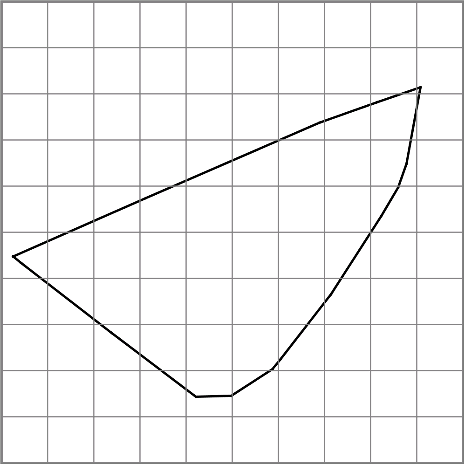2)

```seed = 3
M = 143
9 vertices:
1.197 5.487
1.598 1.427
3.02 1.139
5.11 2.574
8.409 5.388
8.318 7.686
5.059 8.698
1.528 9.296
0.953 9.261

polygon area = 42.371
polygon perimeter = 26.052```3)

```seed = 4
M = 283
11 vertices:
1.739 5.856
2.405 9.504
2.291 9.735
2.001 9.967
1.636 9.453
1.056 8.635
0.946 7.956
0.689 4.268
0.607 2.587
0.718 1.255
0.898 1.76

polygon area = 6.702
polygon perimeter = 18.092```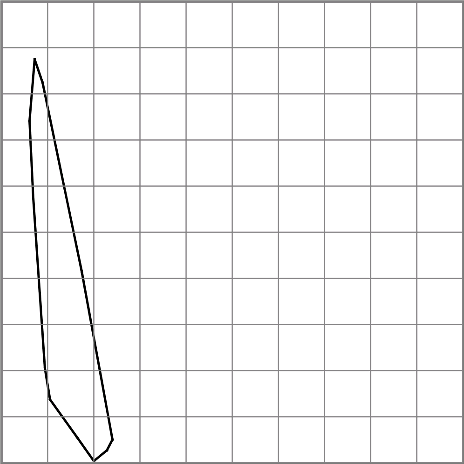4)

```seed = 5
M = 207
5 vertices:
0.486 7.971
3.578 8.536
9.49 7.914
4.504 5.3
2.072 4.05

polygon area = 20.302
polygon perimeter = 21.681```5)

```seed = 6
M = 118
8 vertices:
6.192 3.337
7.242 6.17
8.038 9.119
7.742 8.952
6.675 6.623
4.519 1.832
3.842 0.139
4.452 0.405

polygon area = 6.268
polygon perimeter = 20.129```6)

```seed = 7
M = 166
18 vertices:
3.058 5.553
3.866 6.408
6.222 8.787
7.158 9.375
8.385 9.67
9.057 9.252
9.608 7.356
9.463 5.606
9.319 4.602
9.033 3.613
7.538 2.784
6.802 2.456
4.889 1.624
1.087 0.308
0.213 0.087
0.419 0.539
2.294 4.626
2.538 5

polygon area = 45.368
polygon perimeter = 29.182```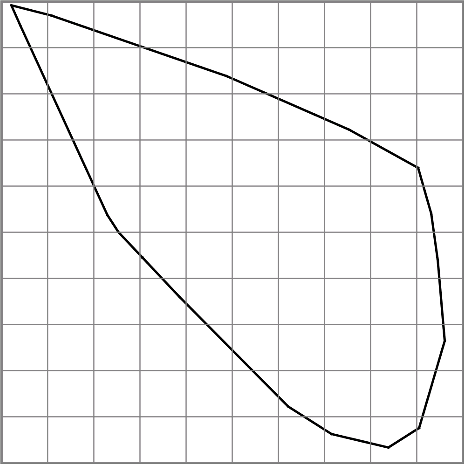7)

```seed = 8
M = 254
14 vertices:
5.06 2.085
4.58 0.441
4.214 0.338
2.569 2.595
2.252 3.373
1.759 4.66
1.231 8.003
1.366 9.274
1.525 9.422
2.562 9.29
5.252 8.79
5.206 4.622
5.181 3.494
5.124 2.416

polygon area = 26.521
polygon perimeter = 22.479```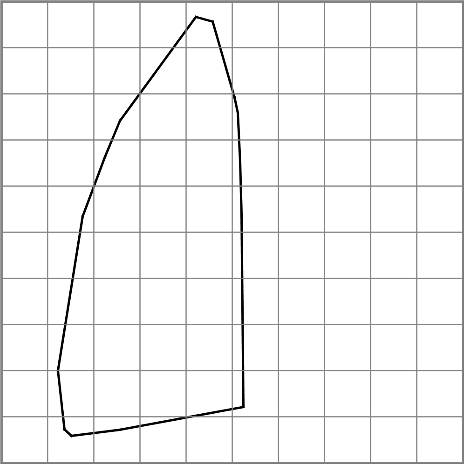8)

```seed = 9
M = 281
7 vertices:
8.32 8.889
2.431 5.661
0.335 2.053
0.843 0.963
5.807 4.079
6.498 4.533
8.733 6.431

polygon area = 25.582
polygon perimeter = 24.202```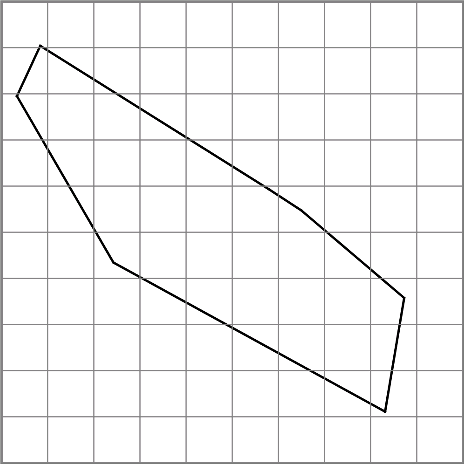9)

```seed = 10
M = 191
5 vertices:
0.214 8.529
7.193 1.607
9.148 0.562
8.389 5.191
0.277 9.317

polygon area = 24.101
polygon perimeter = 26.629```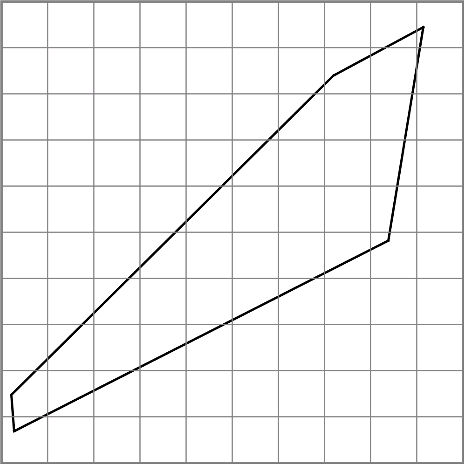This problem statement is the exclusive and proprietary property of TopCoder, Inc. Any unauthorized use or reproduction of this information without the prior written consent of TopCoder, Inc. is strictly prohibited. (c)2010, TopCoder, Inc. All rights reserved.